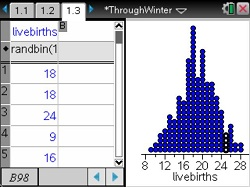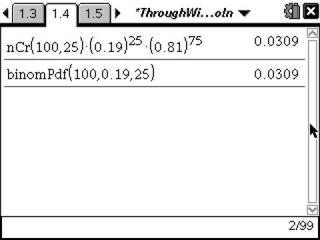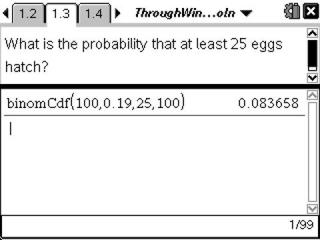# Activities

••• ##### Subject Area

• Math: Statistics: Normal Distributions
• Math: Statistics: Probability and Random Variables

• ##### Author9-12

20 Minutes

• ##### Device
•TI-Nspire™ CX/CX II
•TI-Nspire™ CX CAS/CX II CAS
• TI-Nspire™ Navigator™
• TI-Nspire™
• TI-Nspire™ CAS
• ##### Software

TI-Nspire™
TI-Nspire™ CAS

3.0

• ##### Report an Issue

Makin' It Through The Winter#### Activity Overview

Students simulate a binomial distribution and calculate probabilities for a variety of situations involving binomial probability distributions.

#### Key Steps

•Students begin by investigating a binomial distribution by using a simulation. They create a list of 200 trials using the Random Binomial command, effectively simulating data with a given probability of success. Then, students will graph the data with a quick graph to determine the experimental probability.

•Students calculate theoretical probabilities of a single event using the formula and the built-in handheld command, Binomial Pdf.

•Students calculate theoretical probabilities for ‘at least’ and ‘at most’ of a specific x-value using the built-in handheld command, Binomial Cdf.